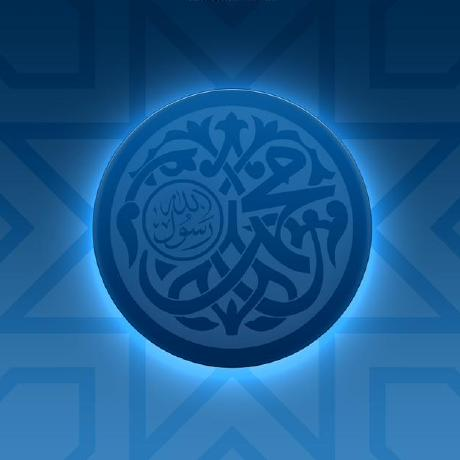home machine learning project : identifying cutlery items

machine learningmachine learning is becoming crucial to add to your backpack. this post attempts to show a basic application of machine learning. we need to determine whether some cutlery items are bowls or mugs or plates. this is a practical demonstration of applied theory machine learning is just applied maths ======================================

from start to finish, machine learning is just statistics, equations, calculations and repetitions. you just code an algorithm. tensors are just big words for matrices. the project ===========

we are identifying items from my kitchen so that when presented with an unidentified cutlery, we can attempt to classify it characteristics of our target

we will be identifying plates, mugs and bowls. here is an overview of their common characteristics

[caption id=”attachment_147” align=”aligncenter” width=”300”]target data[/caption] our collected data

here is our measurement sheet, it could have been a spreadsheet. heights in cm

[caption id=”attachment_148” align=”aligncenter” width=”225”]our data[/caption]

we reformat it into cutlery.csv as follows :

``````D,h,type
17,5,bowl
15.5,5,bowl
22.5,1,plate
8,7.5,mug
8,9,mug
6,11,mug
6,10,mug
24,2.5,plate
26,2,plate
11,8,mug
18,5.5,bowl
14,8,bowl
``````

## reformatting for calculations : plotting

let us plot our data :

[caption id=”attachment_149” align=”aligncenter” width=”1277”]cutlery Jupyter[/caption]

now to select the entire D column we do :

``````df['D']
``````

outputs :

``````0     17.0
1     15.5
2     22.5
3      8.0
4      8.0
5      6.0
6      6.0
7     24.0
8     26.0
9     11.0
10    18.0
11    14.0
Name: D, dtype: float64
``````

same for df[‘h’] viewing our data ================

code :

``````import pandas as pd
import matplotlib.pyplot as plt

# df is short for dataframe

D = df['D']
h = df['h']

for i,type_ in enumerate(df['type']):
D_ = D[i]
h_ = h[i]
if type_ == 'bowl':
plt.scatter(D_, h_, marker='o', color='red', label='a')
plt.text(D_+0.3, h_+0.3, type_, fontsize=9)
elif type_ == 'mug':
plt.scatter(D_, h_, marker='o', color='blue')
plt.text(D_+0.3, h_+0.3, type_, fontsize=9)
elif type_ == 'plate':
plt.scatter(D_, h_, marker='o', color='green')
plt.text(D_+0.3, h_+0.3, type_, fontsize=9)

plt.show()
``````

output :

[caption id=”attachment_155” align=”aligncenter” width=”378”]view data[/caption] our sample

now let us say that we have this :

width D : 8 and h : 7.5

[caption id=”attachment_157” align=”aligncenter” width=”512”]cup from kitchen[/caption]

without seeing the image, having D:9 and h:14, how do we guess what type of cutlery it is? guess by distance

since this is but points on graph, we’ll measure the distance between D:9 and h:14 i.e. (9,14) to each point

we’ll use the simple distance formula :

distance = square_root(  ( X2-X1)^2  +  (Y2-Y1)^2 )

code :

``````import pandas as pd
import matplotlib.pyplot as plt
from math import sqrt

# df is short for dataframe

D = df['D']
h = df['h']

target = (8, 7.5) # tuple
Dt = target # Dt for D-target
ht = target # ht for h-target

plt.figure(figsize=(14,5)) # size of plot in inches

for i,type_ in enumerate(df['type']): # i list for index type\_ for list element
D_ = D[i]
h_ = h[i]
dist = sqrt( (Dt-D_)**2 + (ht-h_)**2 ) # formula

label = '{} \ndist:{}'.format(type_, round(dist, 2))
if type_ == 'bowl':
plt.scatter(D_, h_, marker='o', color='red')
plt.text(D_+0.3, h_, label, fontsize=9)
elif type_ == 'mug':
plt.scatter(D_, h_, marker='o', color='blue')
plt.text(D_+0.3, h_, label, fontsize=9)
elif type_ == 'plate':
plt.scatter(D_, h_, marker='o', color='green')
plt.text(D_+0.3, h_, label, fontsize=9)

plt.scatter(Dt, ht, marker='x', color='green') # target point

plt.annotate('target',
ha = 'center', va = 'bottom',
xytext = (Dt-2.5, ht-2),
xy = (Dt, ht),
arrowprops = { 'facecolor' : 'green', 'shrink' : 0.05 }) # target arrow
plt.xlabel('diameter')
plt.ylabel('height')
plt.show()
``````

output :

[caption id=”attachment_158” align=”aligncenter” width=”847”]distance to target[/caption]

we’ll see that the distance is nearest to all mug samples i.e. from 1.5 to 4 than it is to the nearest bowl (dist:6.02) or plate (dist:15.89)

so we can say that it is a mug / cup

of course, since we are only calculating dist, we can tweak our code to do everything without graphs another sample

consider the following cup with D:9 and h:14

[caption id=”attachment_156” align=”aligncenter” width=”512”]mug sample[/caption]

we set our target to

``````target = (9, 14) # tuple

``````

and our output is :

[caption id=”attachment_159” align=”aligncenter” width=”847”]sample two graph[/caption] machine learning concept applied ================================

this is a k-nearest neighbour application which is labelled under classification aka the basic of machine learning.

learning type: supervised learning

this was a demo project normally much more data has to be collected ! about the title

i wanted to put up something that would not scare beginners off. my first title was :

identifying home cutlery items with a k-nearest neighbours inspired method

but no uninitiated would probably want to click on the link!